# Levi-Civita connection

(diff) ← Older revision | Latest revision (diff) | Newer revision → (diff)

An affine connection on a Riemannian spacethat is a Riemannian connection (that is, a connection with respect to which the metric tensor is covariantly constant) and has zero torsion. An affine connection onis determined uniquely by these conditions, hence every Riemannian spacehas a unique Levi-Civita connection. This concept first arose in 1917 with T. Levi-Civita  as the concept of parallel displacement of a vector in Riemannian geometry. The idea itself goes back to F. Minding, who in 1837 introduced the concept of the involute of a curve on a surface.

With respect to a local coordinate system in, where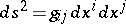, the Levi-Civita connection onis defined by the forms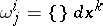, where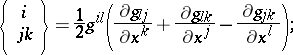its curvature tensor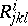is defined by the formula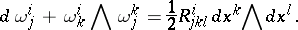Let; then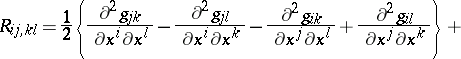thus:The curvature tensor of the Levi-Civita connection hasessential components, where. For example, forthere is only one essential component: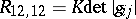, whereis the Gaussian curvature.

If a Riemannian spaceis isometrically immersed in a Euclidean space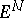, then its Levi-Civita connection is characterized as follows: For two arbitrary vector fields,on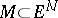the covariant derivative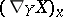at a pointis the orthogonal projection on the tangent plane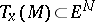of the ordinary differential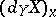of the fieldinwith respect to the vector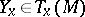. In other words, the mapping of a neighbouring infinitely close tangent plane onto the original tangent plane is accomplished by orthogonal projection.

How to Cite This Entry:
Levi-Civita connection. Encyclopedia of Mathematics. URL: http://encyclopediaofmath.org/index.php?title=Levi-Civita_connection&oldid=14415
This article was adapted from an original article by Ãœ. Lumiste (originator), which appeared in Encyclopedia of Mathematics - ISBN 1402006098. See original article